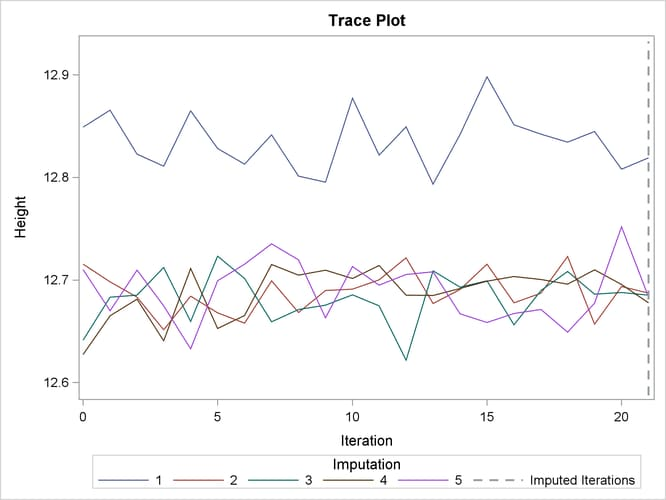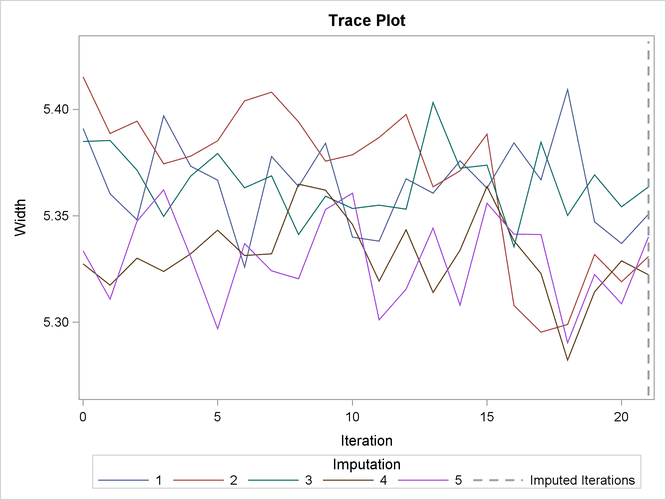### Example 57.8 FCS Method with Trace Plot

This example uses FCS methods to impute missing values in both continuous and classification variables in a data set with an arbitrary missing pattern. The following statements use a logistic regression method to impute values of the classification variable `Species`:

```ods graphics on;
proc mi data=Fish3 seed=1305417 out=outex8;
class Species;
fcs plots=trace
logistic(Species= Height Width Height*Width /details);
var Species Height Width;
run;
ods graphics off;
```

The Model Information  table in Output 57.8.1 describes the method and options used in the multiple imputation process. By default, a regression method is used to impute missing values in each continuous variable.

Output 57.8.1: Model Information

The MI Procedure

Model Information
Data Set WORK.FISH3
Method FCS
Number of Imputations 5
Number of Burn-in Iterations 20
Seed for random number generator 1305417

The FCS Model Specification  table in Output 57.8.2 describes methods and imputed variables in the imputation model. The procedure uses the logistic regression method to impute the variable `Species`, and the regression method to impute variables `Height` and `Width`.

Output 57.8.2: FCS Model Specification

FCS Model Specification
Method Imputed Variables
Regression Height Width
Logistic Regression Species

The Missing Data Patterns table in Output 57.8.3 lists distinct missing data patterns with corresponding frequencies and percentages.

Output 57.8.3: Missing Data Patterns

Missing Data Patterns
Group Species Height Width Freq Percent Group Means
Height Width
1 X X X 40 76.92 12.627350 5.347450
2 X X . 1 1.92 18.037000 .
3 . X X 3 5.77 11.797667 4.587667
4 . X . 4 7.69 13.346750 .
5 . . X 2 3.85 . 5.135000
6 O O O 2 3.85 . .

When you use the DETAILS keyword in the LOGISTIC option, parameters estimated from the observed data and the parameters used in each imputation are displayed in the Logistic Models for FCS Method table in Output 57.8.4.

Output 57.8.4: FCS Logistic Regression Model for `Species`

Logistic Models for FCS Method
Imputed
Variable
Effect Imputation
1 2 3 4 5
Species Intercept 27.250540 27.240843 27.242699 27.254682 27.251468
Species Height 59.970974 59.962249 59.964027 59.980855 59.971742
Species Width -26.032805 -26.021304 -26.023556 -26.028866 -26.033865
Species Height*Width -6.760308 -6.739118 -6.742934 -6.754780 -6.762477

With ODS Graphics enabled, the PLOTS=TRACE option displays trace plots of means for all continuous variables by default, as shown in Output 57.8.5 and Output 57.8.6. The dashed vertical lines indicate the imputed iterations—that is, the variable values used in the imputations. The plot shows no apparent trends for the two variables.

Output 57.8.5: Trace Plot for `Height`Output 57.8.6: Trace Plot for `Width`The following statements list the first 10 observations of the data set `outex8` in Output 57.8.7:

```proc print data=outex8(obs=10);
title 'First 10 Observations of the Imputed Data Set';
run;
```

Output 57.8.7: Imputed Data Set

 First 10 Observations of the Imputed Data Set

Obs _Imputation_ Species Length Height Width
1 1 Bream 30.0000 11.5200 4.02000
2 1 Bream 31.2000 12.4800 4.30600
3 1 Bream 31.1000 12.3780 4.69600
4 1 Bream 33.5000 12.7300 4.45600
5 1 Pike 68.0455 12.4440 6.46920
6 1 Bream 34.7000 13.6020 4.92700
7 1 Bream 34.5000 14.1800 5.27900
8 1 Pike 35.0000 4.9290 4.69000
9 1 Bream 35.1000 14.0050 4.84400
10 1 Bream 36.2000 14.2270 4.95900

After the completion of five imputations by default, the Variance Information table in Output 57.8.8 displays the between-imputation variance, within-imputation variance, and total variance for combining complete-data inferences for continuous variables. The relative increase in variance due to missingness, the fraction of missing information, and the relative efficiency for each variable are also displayed. These statistics are described in the section Combining Inferences from Multiply Imputed Data Sets.

Output 57.8.8: Variance Information

Variance Information
Variable Variance DF Relative
Increase
in Variance
Fraction
Missing
Information
Relative
Efficiency
Between Within Total
Height 0.003691 0.318783 0.323212 46.369 0.013894 0.013797 0.997248
Width 0.001464 0.017777 0.019534 39.458 0.098807 0.093580 0.981628

The Parameter Estimates table in Output 57.8.9 displays a 95% mean confidence interval and a t statistic with its associated p-value for each of the hypotheses requested with the default MU0=0 option.

Output 57.8.9: Parameter Estimates

Parameter Estimates
Variable Mean Std Error 95% Confidence Limits DF Minimum Maximum Mu0 t for H0:
Mean=Mu0
Pr > |t|
Height 12.710577 0.568517 11.56646 13.85470 46.369 12.677842 12.819080 0 22.36 <.0001
Width 5.331652 0.139764 5.04906 5.61425 39.458 5.287861 5.393360 0 38.15 <.0001